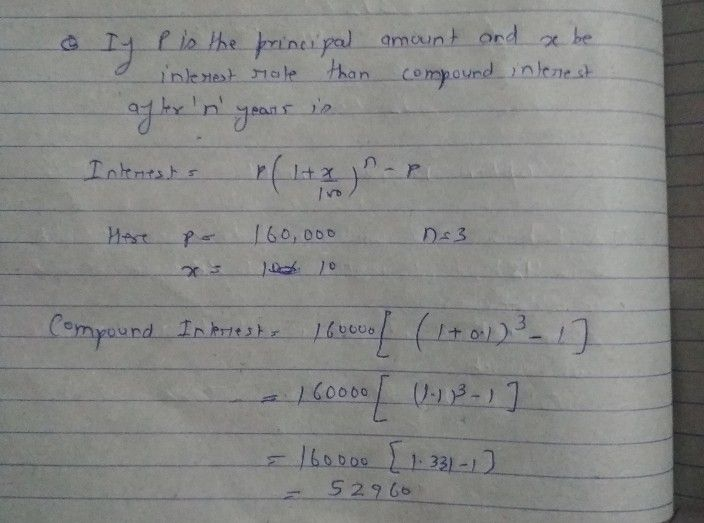Symbol
Problem(a) *1500 for 2 years at 10% per annum compounded annually. (b) 600 for3 years at 10% per annum conmpounded annually. (c) *8500 at 8% per annum for 2 years compounded annually. 15625 at 4% per annum for 3 years compounded annually. (e) 4000 for 2 years at 124% per annum. Salman deposited 18000 with a company at 18% per annum compound interest for 2 years, Calculate the amoun He gets after 2 years. Vasu received a sum of 5000 as a loan from the bank. If the rate of interest is 5%% per annum compounded annuanty, calculate the compound interest that Vasu pays after 2 years. To renovate his house, Mohan obtained a loan of 160,000 from a finance company. If the rate of interest is 10% per annum compounded annually, calculate the compound interest Mohan will have to pay after 3 years. annum for 3 years and the interest is compounded annually. Calculate Sonia invests $10.000c$ at 12% per (i) the amount standing to her credit at the end of second year. the interest for the third year.
7th-9th grade
Other
Search count: 120
SolutionQanda teacher - dushyanthope it will help you